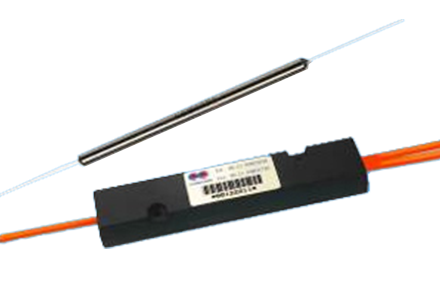### Current Position：Home / Products

 High Power 1x2(2x2) Single Mode Broadband SplitterProduct Features Product Applications Low PDL Optical Communication System Low Insertion Loss Optical Testing System High Power Endured Fiber Optic Sensor Stable and Reliable Optical Power Distributor
 Specifications Splitting Ratio:50:50 Port Configuration Unit 1x2 or 2x2 Bandwidth nm ±40 Insertion Loss Max dB 3.4 Excess Loss Typ dB 0.05 Uniformity Max dB 0.6 PDL Max nm 0.1 Operating Power Min dB 5 Max dB 10 Operating Temperature dB -40 to +85 Storage Temperature W -50 to +85 Package Type mm S11=Ø4x60: for bare fiber S12=Ø4x70: for 0.9mm loose tube M1=9x16x90: for 0.9mm loose tube or 2mm cable or 3mm cable

*Test at central wavelength only. There would be an unused termination port around 20cm for 1x2 version.

 Splitting Ratio & Insertion Loss Conversion Table Splitting Ratio Maximum Insertion Loss (dB) Output Port 1 Output Port 2 50:50 3.4 3.4 60:40 2.5 4.4 70:30 1.8 5.6 80:20 1.1 7.4 90:10 0.6 10.8 95:5 0.4 14.6 96:4 0.3 16.0 97:3 0.3 17.5 98:2 0.2 19.0 99:1 0.2 21.5 99.5:0.5 0.2 23.0
 Ordering Information H P B S Wavelength 1=1625nm 2=1590nm 3=1570nm 4=1550nm 5=1480nm 6=1475nm 7=1310nm P=2000nm S=Specify Structure 1=1x2 2=2x2 Splitting Ratio 05=99.5:0.5 99=99:1 98=98:2 97=97:3 96=96:4 95=95:5 … 50=50:50 Package A=S11 B=S12 D=M1 Fiber Type 1=G652 or Equivalent H=SM1950 Pigtail S=250μm bare fiber M=0.9mm loose tube L=3mm cable R=2mm cable Fiber Length 0=0.5m 1=0.75m 2=1.0m 3=1.5m 4=2.0m S=Specify Connector 0=None 1=FC/PC 2=FC/SPC 3=FC/APC 4=SC/SPC 5=SC/APC 6=ST 7=FC/UPC 8=SC/UPC 9=MU A=LC/PC B=SC/PC C=LC/UPC D=LC/APC

Note:1. Central wavelength can be customized for different applications.
2. All specifications are before connectors and are subject to change without notice.
3. All data are measured at central wavelength at room temperature.